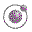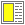Expressions, Types, and Functions - slide 33 : 46

The function concept
The conceptual starting point is the well-known mathematical concept of functions

The notational starting point is lambda calculus

• The mathematical function concept

• A mapping from a domain to a range

• A function transfers values from the domain to values in the range

• A value in the domain has at most a single corresponding value in the range

• Totally or partially defined functions

• Extensionally or intensionally defined functions

• Lambda calculus

• A very terse notation of functions and function application